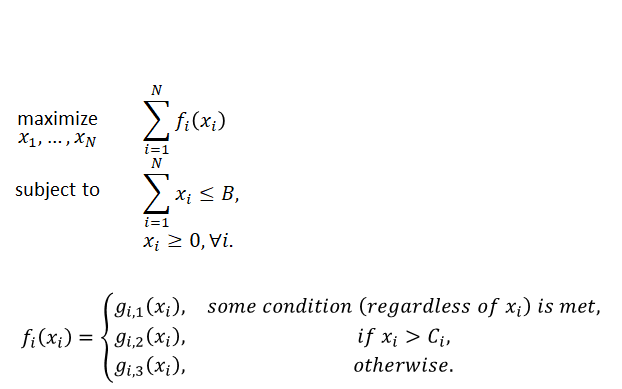# How can I maximize a piecewise concave function?

Hi, I am struggling to construct a code that solves a convex problem.
The problem is a maximization problem with an objective function which is continuous, smooth, and concave, but piecewised.
The problem is as follows:In the above problem, B is a given constant, and C_i for all i are also given constants.
g_{i,1}(x), g_{i,2}(x), g_{i,3}(x) are all concavely increasing function with respect to x.
Also at x=C_i, g_{i,2} and g_{i,3} have the same values and the same derivatives, i.e., g_{i,2}(C_i)=g_{i,3}(C_i) and g_{i,2}’(C_i)=g_{i,3}’(C_i).

How can I solve this convex problem using CVX?

You cannot do that for an arbitrary function f. Therefore, you must have an explicit definition of the g functions.

Also

https://docs.mosek.com/modeling-cookbook/practical.html?highlight=piece#convex-univariate-piecewise-defined-functions

might be useful.

1 Like

Actually functions, g, are explicitly given for me. So, I made a code with them, but the condition (xi>Ci) makes an error. Thank you for link, I will read it.

What I know for sure is that strict greater than is not possible.

Also I doubt you can formulate a function branches. You should be able to do with and that is why I made a reference to Mosek modelling cook book.

Finally showing you Cvx code might be useful because we have no clue what you did.

1 Like

My code that causes the error is as follows:

``````Uset = func1(...); % given
Wset = func2(...); % given
Hset = func3(...); % given

cvx_precision best
cvx_begin quiet
variable x(N) nonnegative

sumF = 0;
for i = 1:N
u1 = Uset1(i);    u2 = Uset2(i);
w1 = Wset1(i);    w2 = Wset2(i);
h1 = Hset1(i);    h2 = Hset2(i);
if w2/w1 <= h1/h2
sumF = sumF + log(1+h1*x(i));
elseif x(i) >= (w1/h2-w2/h1) / (w2-w1)
sumF = sumF + w2*log(1+h2*x(i));
else
sumF = sumF + w1*log(1+h1*cvxp(k))...
+ w1*log((w2-w1)/(h1-h2)*h2/w1)...
+ w2*log((h1-h2)/(w2-w1)*w2/h1);
end
end

maximize sumF
subject to
sum(x) <= 50
cvx_end
``````

Then, I have an error message as follows:

Disciplined convex programming error:
Constraints may not appear in if/then statements.

I guess that the error occurs because `elseif ~~~~~` phrase.
So, I am thinking how to fix it.

You can’t just express your function using if-else where the if-else conditions include values of optimization variables. You would have to model it carefully using binary variables as in the link you got from @Erling or some variation of that. There are resources on mixed-integer modeling of piecewise defined functions online.

1 Like